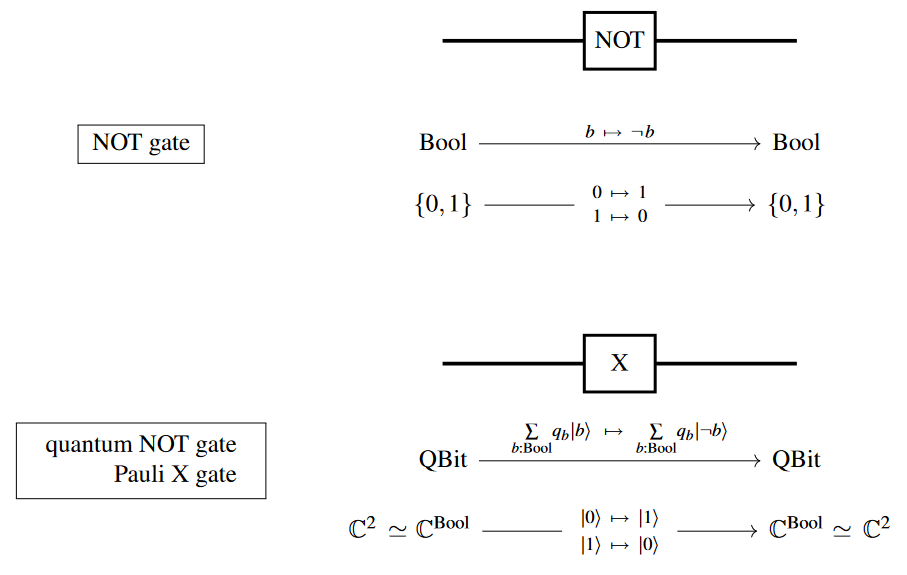Contents

# Contents

## Idea

In (classical) logic, the negation of a statement $p$ is a statement $\neg{p}$ which is true if and only if $p$ is false. Hence, viewed algebraically, the negation corresponds to the complement operator of the corresponding Boolean algebra which satisfies $a\wedge\neg a=\bot$ as well as $a\vee \neg a=\top$.

More generally, as different logics correspond to different types of lattices, one calls negation antitone, or polarity reversing, lattice operators that mimic or approximate the algebraic and proof-theoretic behavior of $\neg$.

## As a logic gate

As a logic gate on bits and as a quantum logic gate on qbits ($X$-Pauli matrix):## Negation in different logics

In classical logic, we have the double negation law:

$\neg\neg{p} \;\equiv\; p \,.$

In intuitionistic logic, we only have

$\neg\neg{p} \;\dashv\; p \,,$

while in paraconsistent logic, we instead have

$\neg\neg{p} \;\vdash\; p \,.$

One may interpret intuitionistic negation as ‘denial’ and paraconsistent negation as ‘doubt’. So when one says that one doesn't deny $p$, that's weaker than actually asserting $p$; while when one says that one doesn't doubt $p$, that's stronger than merely asserting $p$. Paraconsistent logic has even been applied to the theory of law: if $p$ is a judgment that normally requires only the preponderance of evidence, then $\neg\neg{p}$ is a judgment of $p$ beyond reasonable doubt.

Linear logic features (at least) three different forms of negation, one for each of the above. (The default meaning of the term ‘negation’ in linear logic, $p^\bot$, is the one that satisfies the classical double-negation law.)

Accordingly, negation mediates de Morgan duality in classical and linear logic but not in intuitionistic or paraconsistent logic.

## In type theory syntax

In usual type theory syntax negation is obtained as the function type into the empty type: $\not a = a \to \varnothing$.

Equivalently, in type theory with equivalence types but without function types, negation is the equivalence type with the empty type: $\not a = a \simeq \varnothing$.

## In categorical semantics

The categorical semantics of negation is the internal hom into the initial object: $\not = [-, \emptyset]$.

In a topos, the negation of an object $A$ (a proposition under the propositions as types-interpretation) is the internal hom object $0^A$, where $0 = \emptyset$ denotes the initial object.

This matches the intuitionistic notion of negation in that there is a natural morphism $A \to 0^{0^A}$ but not the other way around.

$\phantom{-}$symbol$\phantom{-}$$\phantom{-}$in logic$\phantom{-}$
$\phantom{A}$$\in$$\phantom{A}$element relation
$\phantom{A}$$\,:$$\phantom{A}$typing relation
$\phantom{A}$$=$$\phantom{A}$equality
$\phantom{A}$$\vdash$$\phantom{A}$$\phantom{A}$entailment / sequent$\phantom{A}$
$\phantom{A}$$\top$$\phantom{A}$$\phantom{A}$true / top$\phantom{A}$
$\phantom{A}$$\bot$$\phantom{A}$$\phantom{A}$false / bottom$\phantom{A}$
$\phantom{A}$$\Rightarrow$$\phantom{A}$implication
$\phantom{A}$$\Leftrightarrow$$\phantom{A}$logical equivalence
$\phantom{A}$$\not$$\phantom{A}$negation
$\phantom{A}$$\neq$$\phantom{A}$negation of equality / apartness$\phantom{A}$
$\phantom{A}$$\notin$$\phantom{A}$negation of element relation $\phantom{A}$
$\phantom{A}$$\not \not$$\phantom{A}$negation of negation$\phantom{A}$
$\phantom{A}$$\exists$$\phantom{A}$existential quantification$\phantom{A}$
$\phantom{A}$$\forall$$\phantom{A}$universal quantification$\phantom{A}$
$\phantom{A}$$\wedge$$\phantom{A}$logical conjunction
$\phantom{A}$$\vee$$\phantom{A}$logical disjunction
symbolin type theory (propositions as types)
$\phantom{A}$$\to$$\phantom{A}$function type (implication)
$\phantom{A}$$\times$$\phantom{A}$product type (conjunction)
$\phantom{A}$$+$$\phantom{A}$sum type (disjunction)
$\phantom{A}$$0$$\phantom{A}$empty type (false)
$\phantom{A}$$1$$\phantom{A}$unit type (true)
$\phantom{A}$$=$$\phantom{A}$identity type (equality)
$\phantom{A}$$\simeq$$\phantom{A}$equivalence of types (logical equivalence)
$\phantom{A}$$\sum$$\phantom{A}$dependent sum type (existential quantifier)
$\phantom{A}$$\prod$$\phantom{A}$dependent product type (universal quantifier)
symbolin linear logic
$\phantom{A}$$\multimap$$\phantom{A}$$\phantom{A}$linear implication$\phantom{A}$
$\phantom{A}$$\otimes$$\phantom{A}$$\phantom{A}$multiplicative conjunction$\phantom{A}$
$\phantom{A}$$\oplus$$\phantom{A}$$\phantom{A}$additive disjunction$\phantom{A}$
$\phantom{A}$$\&$$\phantom{A}$$\phantom{A}$additive conjunction$\phantom{A}$
$\phantom{A}$$\invamp$$\phantom{A}$$\phantom{A}$multiplicative disjunction$\phantom{A}$
$\phantom{A}$$\;!$$\phantom{A}$$\phantom{A}$exponential conjunction$\phantom{A}$
• Y. Gauthier, A Theory of Local Negation: The Model and some Applications , Arch. Math. Logik 25 (1985) pp.127-143. (gdz)

• H. Wansing, Negation , pp.415-436 in Goble (ed.), The Blackwell Guide to Philosophical Logic , Blackwell Oxford 2001.